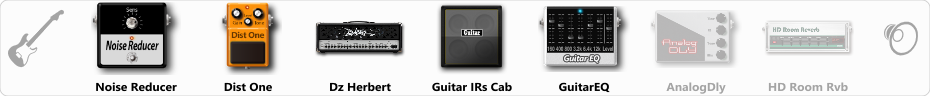# Metal Tone

Discussion in 'ToneLib-GFX presets' started by Savandil, Aug 10, 2019.

1. Metal Tone

Preset name: METAL

Effects chain:Effect: "Noise Reducer" (Dynamics / Filter), active - "yes"
"Sens" = 60
"Mode" = Hard

Effect: "Dist One" (Overdrive / Distortion), active - "yes"
"Drive" = 30
"Tone" = 40
"Level" = 88

Effect: "Dz Herbert" (Amp simulators), active - "yes"
"Gain" = 46
"Bass" = 21
"Middle" = 84
"Treble" = 68
"Presence" = 76
"Master" = 50
"Output" = 50
"Level (dB)" = 0

Effect: "Guitar IRs Cab" (Cabinets), active - "yes"
"Model" = Fender Bassman (2x15")
"Mic Position" = Center
"Mic Distance" = Near
"Low Cut (Hz)" = 60
"Hi Cut (kHz)" = 20.0
"Mix" = 100
"Level (dB)" = 0

Effect: "GuitarEQ" (Dynamics / Filter), active - "yes"
"160 Hz" = 0
"400 Hz" = 0
"800 Hz" = -5
"1.6 kHz" = 0
"3.2 kHz" = -2
"6.4 kHz" = 0
"12 kHz" = 0
"Level (dB)" = 0

Effect: "AnalogDly" (Delay), active - "no"
"Time" = 380
"Feedback" = 34
"Tone" = 65
"Mix" = 65

Effect: "HD Room Rvb" (Reverb), active - "no"
"Time" = 1.3
"PreLPF" = 77
"PreDelay" = 60
"HiDamp" = 65
"LoGain" = -3.5
"Mix" = 41

Note: You will need to download and install the ToneLib-GFX software to use the preset.

File size:
611 bytes
Views:
2,989